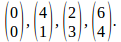## Transformations Using Matrices

Any vector may be operated on my a suitable matrix. Generally the vector is scaled and rotated. If the vectors make up the sides of a polygon then in general the polygon will be stretched or scaled in certain directions and rotated. I will illustrate this below for the unit square.

The corners of the unit square have coordinates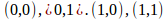We can write these as vectors relative to the origin: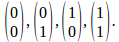Suppose we operate on these vectors with the matrix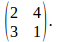The image of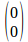is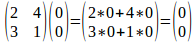The image of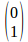is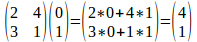The image of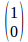is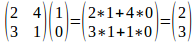The image of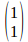is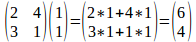The image of the unit squareis